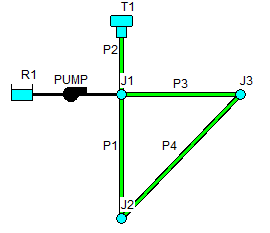OWA-EPANET Toolkit  2.2Network Building Example

This example shows how a network can be built just through toolkit function calls, eliminating the need to always use an EPANET formatted input file. This creates opportunities to use other sources of network data in one's code, such as relational database files or GIS/CAD files.

Below is a schematic of the network to be built.#include "epanet2_2.h"
void netbuilder()
{
// Create a project that uses gpm for flow units and
// the Hazen-Williams formula for head loss
int index;
EN_init(ph, "", "", EN_GPM, EN_HW);
// Add the first junction node to the project with
// an elevation of 700 ft and a demand of 0
EN_setjuncdata(ph, index, 700, 0, "");
// Add the remaining two junctions with elevations of
// 710 ft and demands of 250 and 500 gpm, respectively
EN_setjuncdata(ph, index, 710, 250, "");
EN_setjuncdata(ph, index, 710, 500, "");
// Add the reservoir at an elevation of 650 ft
EN_setnodevalue(ph, index, EN_ELEVATION, 650);
// Add the tank node at elevation of 850 ft, initial water level
// at 120 ft, minimum level at 100 ft, maximum level at 150 ft
// and a diameter of 50.5 ft
EN_settankdata(ph, index, 850, 120, 100, 150, 50.5, 0, "");
// Add the pipes to the project, setting their length,
// diameter, and roughness values
EN_setpipedata(ph, index, 10560, 12, 100, 0);
EN_setpipedata(ph, index, 5280, 14, 100, 0);
EN_setpipedata(ph, index, 5280, 14, 100, 0);
EN_setpipedata(ph, index, 5280, 14, 100, 0);
// Add a pump to the project
// Create a single point head curve (index = 1) and
// assign it to the pump
EN_setcurvevalue(ph, 1, 1, 1500, 250);
// Save the project for future use
EN_saveinpfile(ph, "example2.inp");
// Delete the project
}
EN_PIPE
Pipe.
Definition: epanet2_enums.h:184
EN_ELEVATION
Elevation.
Definition: epanet2_enums.h:39
Adds a new data curve to a project.
EN_setjuncdata
int EN_setjuncdata(EN_Project ph, int index, double elev, double dmnd, char *dmndpat)
Sets a group of properties for a junction node.
EN_saveinpfile
int EN_saveinpfile(EN_Project ph, const char *filename)
Saves a project's data to an EPANET-formatted text file.
EN_RESERVOIR
Reservoir node.
Definition: epanet2_enums.h:174
EN_PUMP_HCURVE
Pump head v. flow curve index.
Definition: epanet2_enums.h:94
int EN_addnode(EN_Project ph, char *id, int nodeType, int *index)
Adds a new node to a project.
EN_setnodevalue
int EN_setnodevalue(EN_Project ph, int index, int property, double value)
Sets a property value for a node.
EN_PUMP
Pump.
Definition: epanet2_enums.h:185
EN_GPM
Gallons per minute.
Definition: epanet2_enums.h:265
EN_Project
struct Project * EN_Project
The EPANET Project wrapper object.
Definition: epanet2_2.h:49
EN_setcurvevalue
int EN_setcurvevalue(EN_Project ph, int curveIndex, int pointIndex, double x, double y)
Sets the value of a single data point for a curve.
EN_createproject
int EN_createproject(EN_Project *ph)
Creates an EPANET project.
EN_TANK
Storage tank node.
Definition: epanet2_enums.h:175
EN_init
int EN_init(EN_Project ph, const char *rptFile, const char *outFile, int unitsType, int headLossType)
Initializes an EPANET project.
EN_HW
Hazen-Williams.
Definition: epanet2_enums.h:251
EN_settankdata
int EN_settankdata(EN_Project ph, int index, double elev, double initlvl, double minlvl, double maxlvl, double diam, double minvol, char *volcurve)
Sets a group of properties for a tank node.
EN_deleteproject
int EN_deleteproject(EN_Project ph)
Deletes a currently opened EPANET project.
epanet2_2.h
EN_JUNCTION
Junction node.
Definition: epanet2_enums.h:173